Main Content

# imwarp

Apply geometric transformation to image

## Syntax

``B = imwarp(A,tform)``
``B = imwarp(A,D)``
``````[B,RB] = imwarp(A,RA,tform)``````
``[___] = imwarp(___,interp)``
``````[___] = imwarp(___,Name,Value)``````

## Description

example

````B = imwarp(A,tform)` transforms the numeric, logical, or categorical image `A` according to the geometric transformation `tform`. The function returns the transformed image in `B`.```
````B = imwarp(A,D)` transforms image `A` according to the displacement field `D`.```
``````[B,RB] = imwarp(A,RA,tform)``` transforms a spatially referenced image specified by the image data `A` and its associated spatial referencing object `RA`. The outputs are a spatially referenced image specified by the image data `B` and its associated spatial referencing object `RB`.```
````[___] = imwarp(___,interp)` specifies the type of interpolation to use.```

example

``````[___] = imwarp(___,Name,Value)``` specifies name-value pair arguments to control various aspects of the geometric transformation. TipIf the input transformation `tform` does not define a forward transform, then use the `OutputView` name-value pair argument to accelerate the transformation. ```

## Examples

collapse all

Read grayscale image into workspace and display it.

```I = imread('cameraman.tif'); imshow(I)```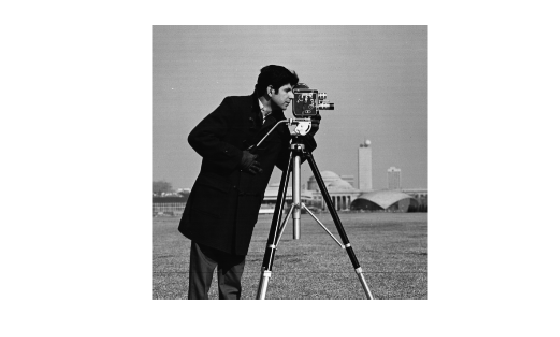Create a 2-D geometric transformation object.

`tform = affine2d([1 0 0; .5 1 0; 0 0 1])`
```tform = affine2d with properties: T: [3x3 double] Dimensionality: 2 ```

Apply the transformation to the image.

```J = imwarp(I,tform); figure imshow(J)```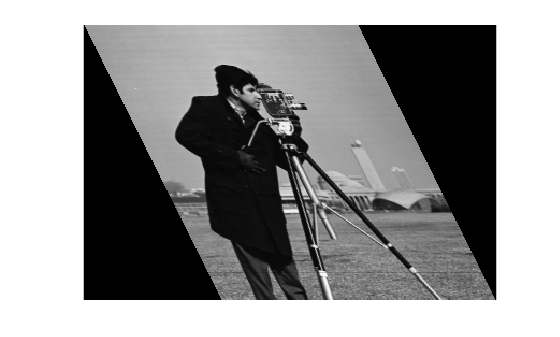Read 3-D MRI data into the workspace and visualize it.

```s = load('mri'); mriVolume = squeeze(s.D); sizeIn = size(mriVolume); hFigOriginal = figure; hAxOriginal = axes; slice(double(mriVolume),sizeIn(2)/2,sizeIn(1)/2,sizeIn(3)/2); grid on, shading interp, colormap gray```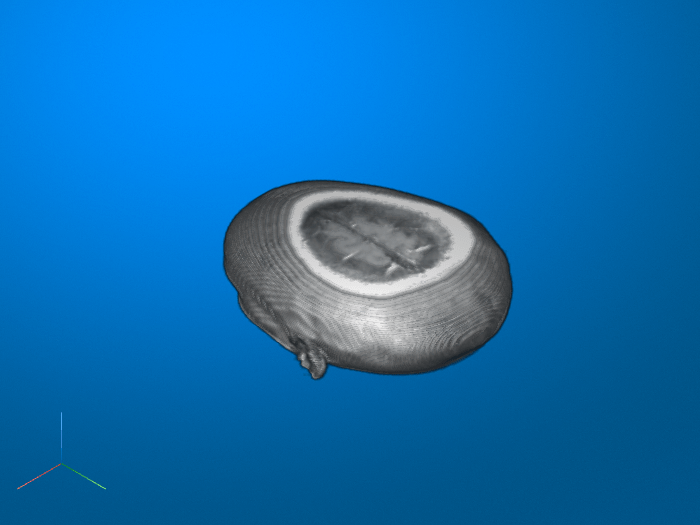Create a 3-D geometric transformation object. First create a transformation matrix that rotates the image around the y-axis. Then create an `affine3d` object from the transformation matrix.

```theta = pi/8; t = [cos(theta) 0 -sin(theta) 0 0 1 0 0 sin(theta) 0 cos(theta) 0 0 0 0 1]; tform = affine3d(t)```
```tform = affine3d with properties: T: [4x4 double] Dimensionality: 3 ```

Apply the transformation to the image.

`mriVolumeRotated = imwarp(mriVolume,tform);`

Visualize three slice planes through the center of the transformed volumes.

```sizeOut = size(mriVolumeRotated); hFigRotated = figure; hAxRotated = axes; slice(double(mriVolumeRotated),sizeOut(2)/2,sizeOut(1)/2,sizeOut(3)/2) grid on, shading interp, colormap gray```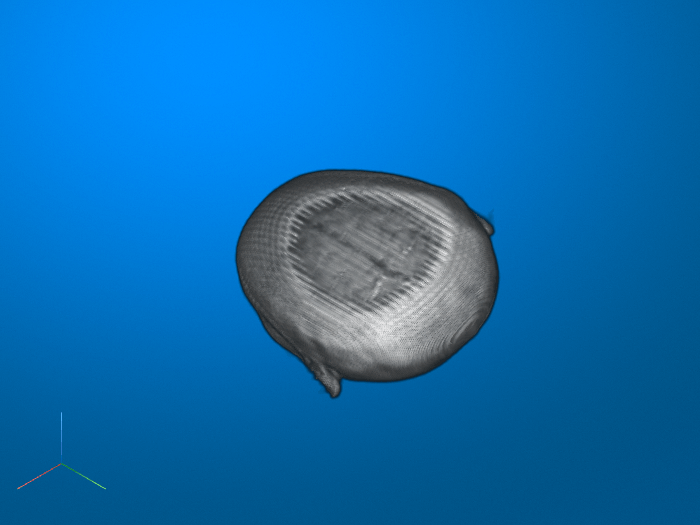Link the views of both axes together.

`linkprop([hAxOriginal,hAxRotated],'View');`

Set the view to see the effect of rotation.

`set(hAxRotated,'View',[-3.5 20.0])`Read and display an image. To see the spatial extents of the image, make the axes visible.

```A = imread('kobi.png'); iptsetpref('ImshowAxesVisible','on') imshow(A)```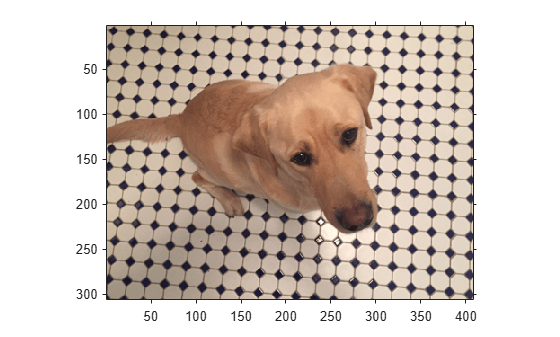Create a 2-D affine transformation. This example creates a randomized transformation that consists of scale by a factor in the range [1.2, 2.4], rotation by an angle in the range [-45, 45] degrees, and horizontal translation by a distance in the range [100, 200] pixels.

`tform = randomAffine2d('Scale',[1.2,2.4],'XTranslation',[100 200],'Rotation',[-45,45]);`

Create three different output views for the image and transformation.

```centerOutput = affineOutputView(size(A),tform,'BoundsStyle','CenterOutput'); followOutput = affineOutputView(size(A),tform,'BoundsStyle','FollowOutput'); sameAsInput = affineOutputView(size(A),tform,'BoundsStyle','SameAsInput');```

Apply the transformation to the input image using each of the different output view styles.

```BCenterOutput = imwarp(A,tform,'OutputView',centerOutput); BFollowOutput = imwarp(A,tform,'OutputView',followOutput); BSameAsInput = imwarp(A,tform,'OutputView',sameAsInput);```

Display the resulting images.

```imshow(BCenterOutput) title('CenterOutput Bounds Style');```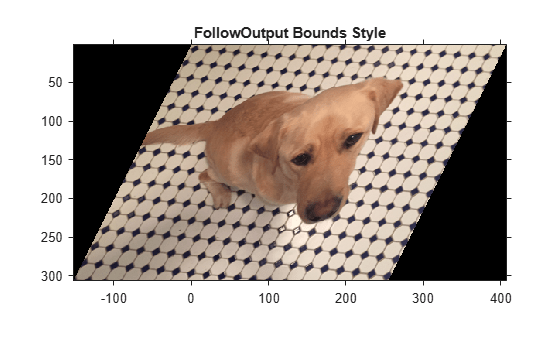```imshow(BFollowOutput) title('FollowOutput Bounds Style');```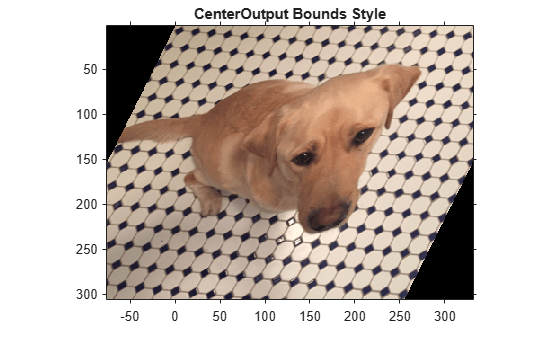```imshow(BSameAsInput) title('SameAsInput Bounds Style');```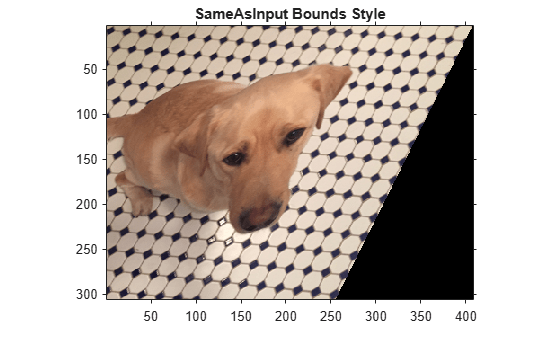`iptsetpref('ImshowAxesVisible','off')`

## Input Arguments

collapse all

Image to be transformed, specified as a numeric, logical, or categorical array of any dimension.

Data Types: `single` | `double` | `int8` | `int16` | `int32` | `int64` | `uint8` | `uint16` | `uint32` | `uint64` | `logical` | `categorical`

Geometric transformation to apply, specified as a `rigid2d`, `affine2d`, `projective2d`, `rigid3d`, or `affine3d` object.

• If `tform` is 2-D and `A` has more than two dimensions, such as for an RGB image, then `imwarp` applies the same 2-D transformation to all 2-D planes along the higher dimensions.

• If `tform` is 3-D, then `A` must be a 3-D image volume.

Displacement field, specified as numeric array. The displacement field defines the grid size and location of the output image. Displacement values are in units of pixels. `imwarp` assumes that `D` is referenced to the default intrinsic coordinate system. To estimate the displacement field, use `imregdemons`.

• If `A` is a 2-D image, then `D` is an m-by-n-by-2 array. The first plane of the displacement field, `D(:,:,1)`, describes the x-component of additive displacement. `imwarp` adds these values to column and row locations in `D` to produce remapped locations in `A`. The second plane of the displacement field, `D(:,:,2)`, describes the y-component of additive displacement values. For 2-D color or multispectral images with multiple color channels, `imwarp` applies the same m-by-n-by-2 displacement field to each channel.

• If `A` is a 3-D image, then `D` is an m-by-n-by-p-by-3 array. The first plane of the displacement field, `D(:,:,1)`, describes the x-component of additive displacement. `imwarp` adds these values to column and row locations in `D` to produce remapped locations in `A`. Similarly, the second and third planes of the displacement field describe the y- and z-component of additive displacement values, respectively.

Data Types: `single` | `double` | `int8` | `int16` | `int32` | `int64` | `uint8` | `uint16` | `uint32` | `uint64`

Spatial referencing information of the image to be transformed, specified as an `imref2d` object for a 2-D transformation or an `imref3d` object for a 3-D transformation.

Type of interpolation used, specified as one of these values.

Interpolation MethodDescription
`'nearest'`

Nearest neighbor interpolation. The output pixel is assigned the value of the pixel that the point falls within. No other pixels are considered.

Nearest-neighbor interpolation is the only method supported for categorical images and it is the default method for images of this type.

`'linear'`Linear interpolation. This is the default interpolation method for numeric and logical images.
`'cubic'`Cubic interpolation

Data Types: `char` | `string`

### Name-Value Pair Arguments

Specify optional comma-separated pairs of `Name,Value` arguments. `Name` is the argument name and `Value` is the corresponding value. `Name` must appear inside quotes. You can specify several name and value pair arguments in any order as `Name1,Value1,...,NameN,ValueN`.

Example: `J = imwarp(I,tform,'FillValues',255)` uses white pixels as fill values.

Size and location of output image in the world coordinate system, specified as the comma-separated pair consisting of `'OutputView'` and an `imref2d` or `imref3d` spatial referencing object. The object has properties that define the size of the output image and the location of the output image in the world coordinate system.

You can create an output view by using the `affineOutputView` function. To replicate the default output view calculated by `imwarp`, use the default bounds style (`'CenterOutput'`) of `affineOutputView`.

You cannot specify `OutputView` when you specify an input displacement field `D`.

Fill values used for output pixels outside the input image, specified as the comma-separated pair consisting of `'FillValues'` and one of the following values. `imwarp` uses fill values for output pixels when the corresponding inverse transformed location in the input image is completely outside the input image boundaries.

The default fill value of numeric and logical images is `0`. The default fill value of categorical images is `missing`, which corresponds to the `<undefined>` category.

Image Type

Transformation Dimensionality

Format of Fill Values

2-D grayscale or logical image2-D
• Numeric scalar

2-D color image or 2-D multispectral image2-D
• Numeric scalar

• c-element numeric vector specifying a fill value for each of the c channels. The number of channels, c, is 3 for color images.

Series of p 2-D images2-D

• Numeric scalar

• c-by-p numeric matrix. The number of channels, c, is 1 for grayscale images and 3 for color images.

N-D image2-D
• Numeric scalar

• Numeric array whose size matches dimensions 3-to-N of the input image `A`. For example, if `A` is 200-by-200-by-10-by-3, then `FillValues` can be a 10-by-3 array.

3-D grayscale or logical image3-D
• Numeric scalar

Categorical image2-D or 3-D
• Valid category in the image, specified as a string scalar or character vector.

• `missing`, which corresponds to the `<undefined>` category. For more information, see `missing`.

Example: `255` fills a `uint8` image with white pixels

Example: `1` fills a `double` image with white pixels

Example: `[0 1 0]` fills a `double` color image with green pixels

Example: `[0 1 0; 0 1 1]'`, for a series of two `double` color images, fills the first image with green pixels and the second image with cyan pixels

Example: `"vehicle"` fills a categorical image with the "vehicle" category

Pad image to create smooth edges, specified as `true` or `false`. When set to `true`, `imwarp` create a smoother edge in the output image by padding the input image with values specified by `FillValues`. When set to `false`, `imwarp` does not pad the image. Choosing `false` (not padding) the input image can result in a sharper edge in the output image. This sharper edge can be useful to minimize seam distortions when registering two images side by side.

## Output Arguments

collapse all

Transformed image, returned as a numeric, logical, or categorical array of the same data type as the input image `A`.

Spatial referencing information of the transformed image, returned as an `imref2d` or `imref3d` spatial referencing object.

## Algorithms

`imwarp` determines the value of pixels in the output image by mapping locations in the output image to the corresponding locations in the input image (inverse mapping). `imwarp` interpolates within the input image to compute the output pixel value.

The following figure illustrates a translation transformation. By convention, the axes in input space are labeled u and v and the axes in output space are labeled x and y. In the figure, note how `imwarp` modifies the spatial coordinates that define the locations of pixels in the input image. The pixel at (1,1) is now positioned at (41,41). In the checkerboard image, each black, white, and gray square is 10 pixels high and 10 pixels wide. For more information about the distinction between spatial coordinates and pixel coordinates, see Image Coordinate Systems.

Input Image Translated## Extended Capabilities

Introduced in R2013a

Download ebook